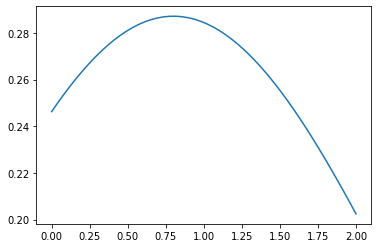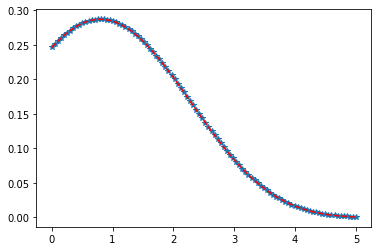# Python – Tukey-Lambda Distribution in Statistics

• Last Updated : 23 Aug, 2021

scipy.stats.tukeylambda() is a Tukey-Lambda continuous random variable. It is inherited from the of generic methods as an instance of the rv_continuous class. It completes the methods with details specific for this particular distribution.
Parameters :

q : lower and upper tail probability
x : quantiles
loc : [optional]location parameter. Default = 0
scale : [optional]scale parameter. Default = 1
size : [tuple of ints, optional] shape or random variates.
moments : [optional] composed of letters [‘mvsk’]; ‘m’ = mean, ‘v’ = variance, ‘s’ = Fisher’s skew and ‘k’ = Fisher’s kurtosis. (default = ‘mv’).
Results : Tukey-Lambda continuous random variable

Attention geek! Strengthen your foundations with the Python Programming Foundation Course and learn the basics.

To begin with, your interview preparations Enhance your Data Structures concepts with the Python DS Course. And to begin with your Machine Learning Journey, join the Machine Learning - Basic Level Course

Code #1 : Creating Tukey-Lambda continuous random variable

## Python3

 `# importing library` `from` `scipy.stats ``import` `tukeylambda``  ` `numargs ``=` `tukeylambda .numargs``a, b ``=` `0.2``, ``0.8``rv ``=` `tukeylambda (a, b)``  ` `print` `(``"RV : \n"``, rv) `

Output :

```RV :
scipy.stats._distn_infrastructure.rv_frozen object at 0x000002A9D9D71F48```

Code #2 : Tukey-Lambda continuous variates and probability distribution

## Python3

 `import` `numpy as np``quantile ``=` `np.arange (``0.01``, ``1``, ``0.1``)` `# Random Variates``R ``=` `tukeylambda .rvs(a, b, size ``=` `10``)``print` `(``"Random Variates : \n"``, R)` `# PDF``x ``=` `np.linspace(tukeylambda.ppf(``0.01``, a, b),``                ``tukeylambda.ppf(``0.99``, a, b), ``10``)``R ``=` `tukeylambda.pdf(x, ``1``, ``3``)``print` `(``"\nProbability Distribution : \n"``, R)`

Output :

```Random Variates :
[ 0.21772132 -0.22664155 -1.59857265  2.60861252  3.14751736  2.06655125
0.62978366  0.28088051 -2.38894301 -1.16725442]

Probability Distribution :
[0.  0.  0.  0.  0.  0.  0.  0.5 0.5 0.5]```

Code #3 : Graphical Representation.

## Python3

 `import` `numpy as np``import` `matplotlib.pyplot as plt``   ` `distribution ``=` `np.linspace(``0``, np.minimum(rv.dist.b, ``3``))``print``(``"Distribution : \n"``, distribution)``   ` `plot ``=` `plt.plot(distribution, rv.pdf(distribution))`

Output :

```Distribution :
[0.         0.04081633 0.08163265 0.12244898 0.16326531 0.20408163
0.24489796 0.28571429 0.32653061 0.36734694 0.40816327 0.44897959
0.48979592 0.53061224 0.57142857 0.6122449  0.65306122 0.69387755
0.73469388 0.7755102  0.81632653 0.85714286 0.89795918 0.93877551
0.97959184 1.02040816 1.06122449 1.10204082 1.14285714 1.18367347
1.2244898  1.26530612 1.30612245 1.34693878 1.3877551  1.42857143
1.46938776 1.51020408 1.55102041 1.59183673 1.63265306 1.67346939
1.71428571 1.75510204 1.79591837 1.83673469 1.87755102 1.91836735
1.95918367 2.        ]
```Code #4 : Varying Positional Arguments

## Python3

 `import` `matplotlib.pyplot as plt``import` `numpy as np` `x ``=` `np.linspace(``0``, ``5``, ``100``)``   ` `# Varying positional arguments``y1 ``=` `tukeylambda.pdf(x, a, b)``y2 ``=` `tukeylambda.pdf(x, a, b)``plt.plot(x, y1, ``"*"``, x, y2, ``"r--"``)`

Output :My Personal Notes arrow_drop_up